Courses

# Heat Effects of Phase Change Civil Engineering (CE) Notes | EduRev

## Civil Engineering (CE) : Heat Effects of Phase Change Civil Engineering (CE) Notes | EduRev

The document Heat Effects of Phase Change Civil Engineering (CE) Notes | EduRev is a part of the Civil Engineering (CE) Course Thermodynamics.
All you need of Civil Engineering (CE) at this link: Civil Engineering (CE)

Heat Effects of Phase Change

A change of phase is generally accompanied by heat release or absorption. The heat effect during the change of phase from liquid to gas, for example, is called the latent heat (or enthalpy of vapourization), while for a transition from soild to liquid the effect is termed heat of fusion. Such enthalpy changes typically quantify the amount of heat need to change the phase of one mole of a substance and are usually obtained experimentally. However, correlations have been developed for their prediction as well. The Reidel equation (L. Riedel, Chem. Ing. Tech., vol. 26, pp. 679-683, 1954) provides a suitable expression for the enthalpy of vapourization at the normal boling point of a pure substance: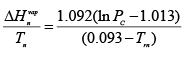...(2.36)

Where, n = normal boiling point, PC

The Watson equation may be used (K. M. Watson, Ind. Eng. Chem., vol. 35, pp. 398-406, 1943) for computing the enthalpy of vaporization of a pure liquid at any temperature using its value at a chosen datum temperature: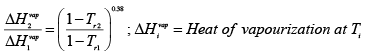...(2.37)

Appendix: Lee-Kesler and Pitzer Methods

Lee Kesler Method: The method used a modified Bendict-Webb-Rubin equation using Pitzer’s acentric factor (ω ). The compressibility factor of a real fluid is related to the properties of a simple fluid (ω= 0) and those of a reference fluid; for the latter n-Octane is used... The compressibility factor Z for the fluid is given by: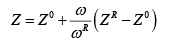Where Z = compressibility factor for the fluid

Z0 = compressibility factor for the simple fluid

ZR= compressibility factor for the reference fluid

ω = acentric factor for the fluid
ω R = acentric factor for the reference fluid (n-octane) = 0.3978.

According to the theory of corresponding states ω = 0, for “simple fluids”: i.e. whose molecules are considered spherical; examples include noble gases, such as argon, krypton and xenon.
Sphericity of a molecule tends to reduce its polarity, as the centre of negative and positive

charges approach each other, leading to low dipole moments. This is also true for relatively linear but symmetric molecule. For example, carbon dioxide has virtually no dipole moment; however, it possesses a quadrupole moment. The acentric factor, therefore, is an indirect measure of the dipole moment of a molecule, and hence of the deviation of the intermolecular potential of real fluids from that of simple fluids.

The compressibility factors for the simple fluid (Z0) and the reference fluid (ZR) are generated from the following equation which is a variant for the BWR EOS (eqn. 2.17):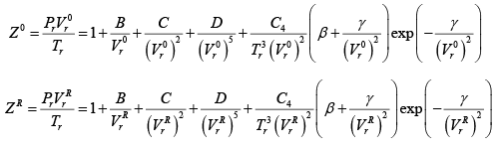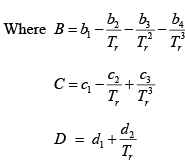...(A.2.4 - A.2.6)

Where Pr and Tr are the reduced pressure and temperature, respectively, at which the volume of the real fluid is required.

Further,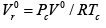...(A.2.7)

Where, V0 = molar volume of the simple fluid (at the given T & P). The Lee-Kesler constants for the simple fluid and the reference fluid to be used with Eqns. 2-6 are enumerated in Table A.1.1 below.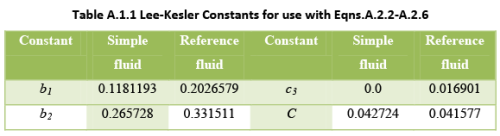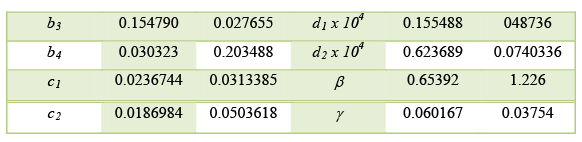The method of calculating the volume at a given Tand Pr
• Step 1: using the critical properties of the real fluid find is as follows: V0r by eqn. A.2.7
• Step 2: using Vr0 , calculate Z
• Step 3: using the given values of Tand Pr  by eqn. A.2.2  is solved again, but with reference fluid constants from above table. The solution obtained for  is now called VrR
• Step 4: ZR, the compressibility for the reference fluid, is next computed with: given by Z = PVrR / Tr                         ....(A.2.8)

• Step 5: With the above values of Z0 and ZR , the compressibility factor Z for the fluid in question can be calculated from Eqn.A.2.1.

The Generalized Lee-Kesler Charts essentially present the values of  Z0  and Z1obtained by the above procedure as function of Tr and Pr A variety of other thermodynamic variables can also be computed in an identical manner. Examples include residual enthalpy and entropy, and gas fugacity. These properties are presented either in the form of figures or charts .

Pitzer Method:

The Pitzer method is nearly identical to that of Lee-Kesler; it assumes that the compressibility factor is linearly dependent on the acentric factor. Thus, eqn. A.2.1 is reformulated using the compressibility factors of both the simple (1) and reference fluid (2), whence: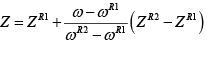...(A.2.9)

Any two fluids may be used as the reference fluids. The method of computing the values of ZR 2 and ZR1 and hence, Z for the fluid of interest follows the same procedure described above for Lee-Kesler.

Offer running on EduRev: Apply code STAYHOME200 to get INR 200 off on our premium plan EduRev Infinity!

## Thermodynamics

56 videos|92 docs|33 tests

,

,

,

,

,

,

,

,

,

,

,

,

,

,

,

,

,

,

,

,

,

;